Search test library by skills or roles
⌘ K

Here are some sample R questions from our premium questions library (10273 non-googleable questions).

### Skills

#### Accounting

🧐 Question

Medium

Applying Functions
Data frames
Functions
Math
Solve
`Consider the following R code:`
`This script defines a function apply_fun that calculates the mean of a vector and adds it to the standard deviation of the vector. The apply function is then used to apply this function to each column of the data frame df. What will be the value of result after the script is run?`

Medium

Dataframe Transform
Solve
`Review the following Dataframe 'bazinga':`
`Which of the following commands would turn it into the Dataframe shown below:`

Medium

Matrix Manipulation
Matrices
Lists
Solve
`Consider the following pseudo code:`
`What will be the state of list_of_matrices after running the script?`
🧐 Question🔧 Skill

Medium

Applying Functions
Data frames
Functions
Math
3 mins
R
Solve

Medium

Dataframe Transform
3 mins
R
Solve

Medium

Matrix Manipulation
Matrices
Lists
3 mins
R
Solve
🧐 Question🔧 Skill💪 Difficulty⌛ Time
Applying Functions
Data frames
Functions
Math
R
Medium3 mins
Solve
Dataframe Transform
R
Medium3 mins
Solve
Matrix Manipulation
Matrices
Lists
R
Medium3 mins
Solve

## Adaface questions are trusted by enterprises globally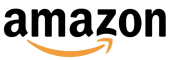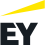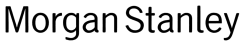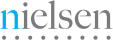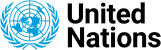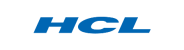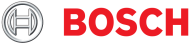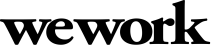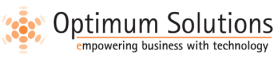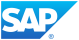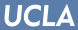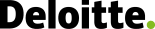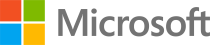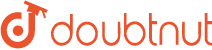We evaluated several of their competitors and found Adaface to be the most compelling. Great default library of questions that are designed to test for fit rather than memorization of algorithms.

Swayam Narain, CTO, AffableJoin 1200+ companies in 75+ countries.
Try the most candidate friendly skills assessment tool today.